Function Repository Resource:

# WeightedDistanceGraph

Given vertices, get a complete graph with edge weights equal to edge lengths

Contributed by: Ed Pegg Jr
 ResourceFunction["WeightedDistanceGraph"][vert] given the list of vertices vert, returns a graph where edges are vertex pairs weighted by their Euclidean distance.

## Examples

### Basic Examples (2)

The output based on the Kreisel–Kurz integral heptagon looks like a random complete graph:

 In:=Out=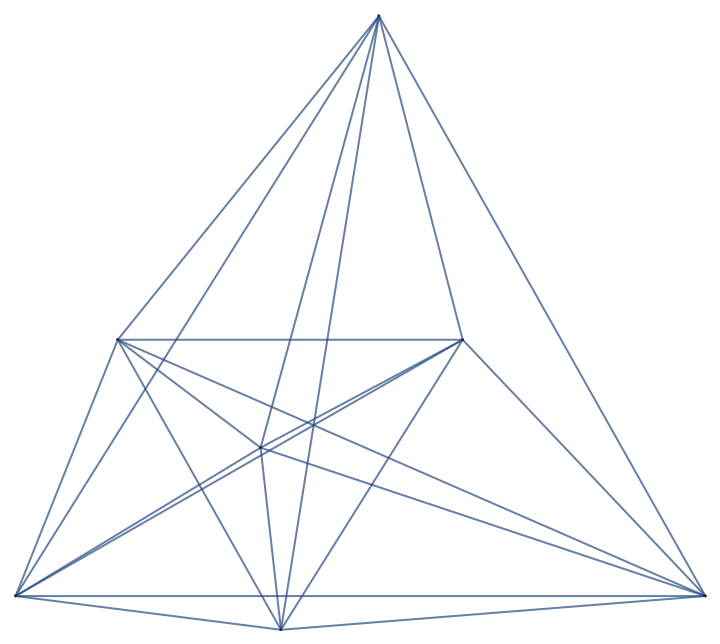The graph has edge weights:

 In:=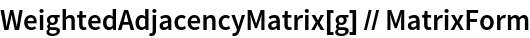Out=### Scope (3)

The Wolfram Language has several graph functions that work on point sets that do not return weighted graphs. Operations on graphs without proper weighting can return unexpected results:

 In:=Out=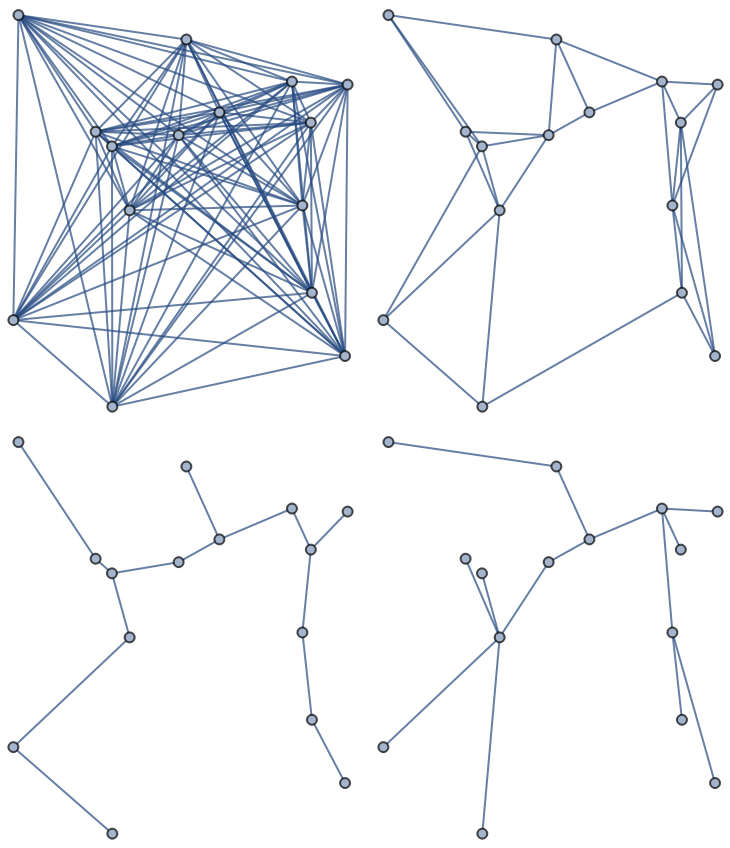With weights given to edges, a method like Kruskal’s algorithm can work as expected:

 In:=Out=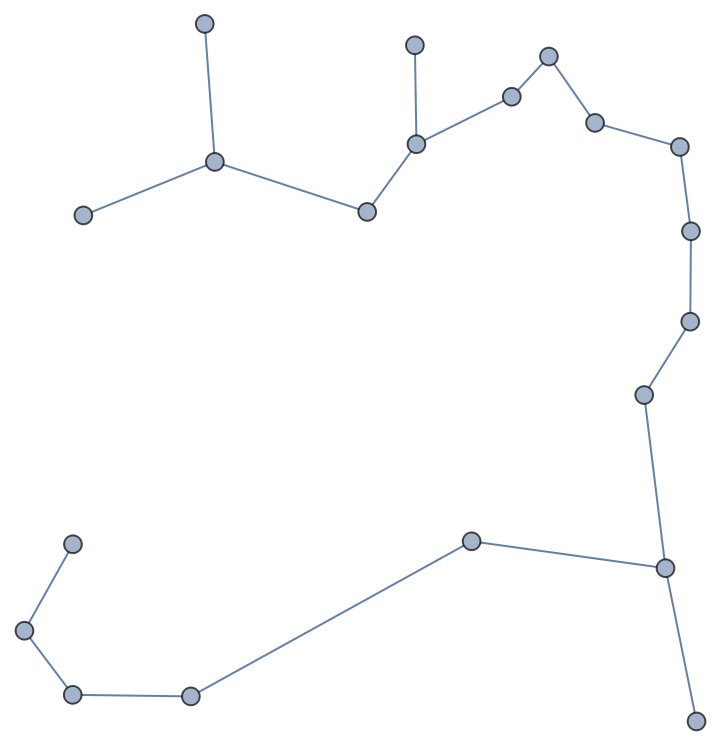With weights given to edges, a method like Prim’s algorithm can work as expected:

 In:=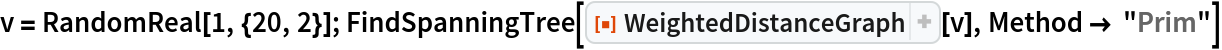Out=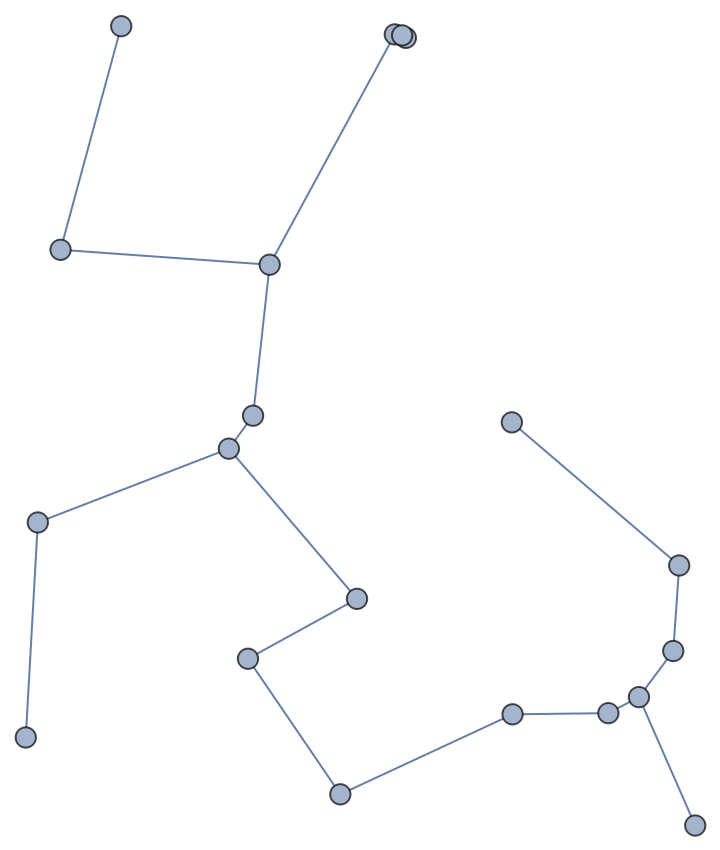### Possible Issues (2)

Using weighted graphs for finding a minimal spanning tree does not scale up well for larger graphs:

 In:=Out=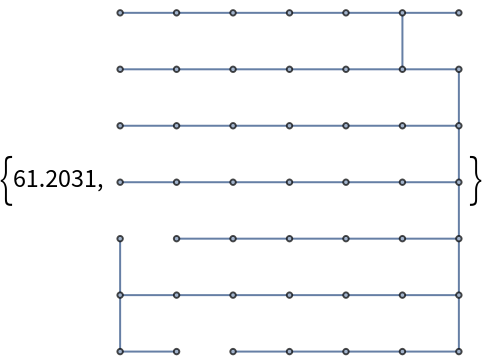In these cases it is better to go right to the algorithm, which is several thousand times faster:

 In:=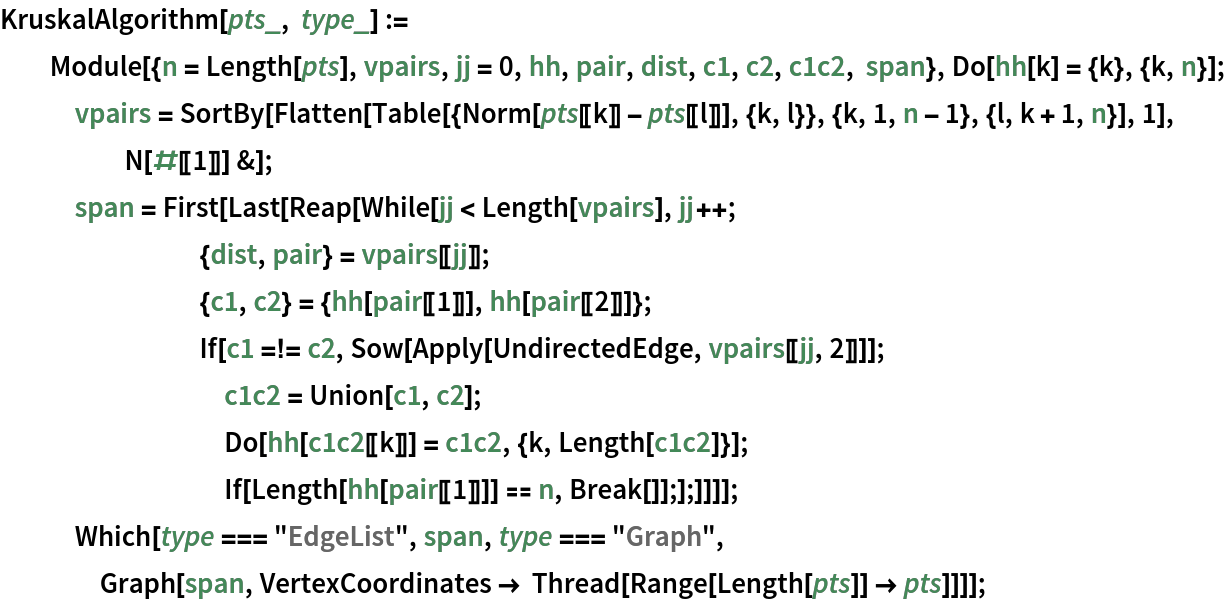In:=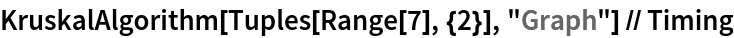Out=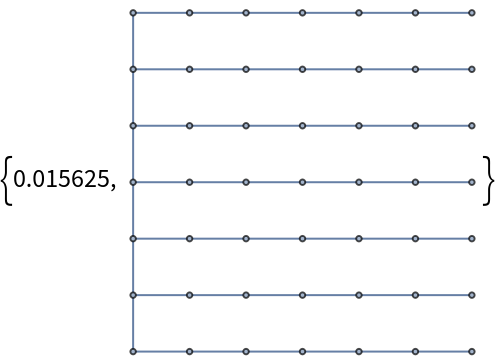## Requirements

Wolfram Language 11.3 (March 2018) or above

## Version History

• 1.0.0 – 15 February 2019